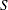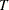### Example 2.3 Linear Assignment Problem for Minimizing Swim Times

A swimming coach needs to assign male and female swimmers to each stroke of a medley relay team. The swimmers’ best times for each stroke are stored in a SAS data set. The LINEAR_ASSIGNMENT statement evaluates the times and matches strokes and swimmers to minimize the total relay swim time.

The data are stored in matrix format, where the row identifier is the swimmer’s name (variable `name`) and each event is a column (variables: `back`, `breast`, `fly`, and `free`). In the following DATA step, the relay times are split into two categories, male and female:

```data RelayTimes;
input name \$ sex \$ back breast fly free;
datalines;
Sue     F 35.1 36.7 28.3 36.1
Karen   F 34.6 32.6 26.9 26.2
Jan     F 31.3 33.9 27.1 31.2
Andrea  F 28.6 34.1 29.1 30.3
Carol   F 32.9 32.2 26.6 24.0
Ellen   F 27.8 32.5 27.8 27.0
Jim     M 26.3 27.6 23.5 22.4
Mike    M 29.0 24.0 27.9 25.4
Sam     M 27.2 33.8 25.2 24.1
Clayton M 27.0 29.2 23.0 21.9
;

data RelayTimesF RelayTimesM;
set RelayTimes;
if      sex='F' then output RelayTimesF;
else if sex='M' then output RelayTimesM;
run;
```

The following statements solve the linear assignment problem for both male and female relay teams:

```proc optnet
data_matrix = RelayTimesF;
linear_assignment
out      = LinearAssignF
id       = (name sex);
run;
%put &_OROPTNET_;
%put &_OROPTNET_LAP_;
```
```proc optnet
data_matrix = RelayTimesM;
linear_assignment
out      = LinearAssignM
id       = (name sex);
run;
%put &_OROPTNET_;
%put &_OROPTNET_LAP_;
```

The progress of the two PROC OPTNET calls is shown in Output 2.3.1 and Output 2.3.2.

Output 2.3.1: PROC OPTNET Log: Linear Assignment for Female Swim Times

 NOTE: ------------------------------------------------------------------------- NOTE: Running OPTNET. NOTE: ------------------------------------------------------------------------- NOTE: The number of columns in the input matrix is 4. NOTE: The number of rows in the input matrix is 6. NOTE: Data input used 0.00 (cpu: 0.00) seconds. NOTE: ------------------------------------------------------------------------- NOTE: Processing LINEAR_ASSIGNMENT statement. NOTE: The minimum cost linear assignment is 111.5. NOTE: Processing the linear assignment problem used 0.00 (cpu: 0.00) seconds. NOTE: ------------------------------------------------------------------------- NOTE: Data output used 0.00 (cpu: 0.00) seconds. NOTE: ------------------------------------------------------------------------- NOTE: The data set WORK.LINEARASSIGNF has 4 observations and 4 variables. STATUS=OK  LAP=OPTIMAL STATUS=OPTIMAL  OBJECTIVE=111.5  CPU_TIME=0.00  REAL_TIME=0.00

Output 2.3.2: PROC OPTNET Log: Linear Assignment for Male Swim Times

 NOTE: ------------------------------------------------------------------------- NOTE: Running OPTNET. NOTE: ------------------------------------------------------------------------- NOTE: The number of columns in the input matrix is 4. NOTE: The number of rows in the input matrix is 4. NOTE: Data input used 0.00 (cpu: 0.00) seconds. NOTE: ------------------------------------------------------------------------- NOTE: Processing LINEAR_ASSIGNMENT statement. NOTE: The minimum cost linear assignment is 96.6. NOTE: Processing the linear assignment problem used 0.00 (cpu: 0.00) seconds. NOTE: ------------------------------------------------------------------------- NOTE: Data output used 0.00 (cpu: 0.00) seconds. NOTE: ------------------------------------------------------------------------- NOTE: The data set WORK.LINEARASSIGNM has 4 observations and 4 variables. STATUS=OK  LAP=OPTIMAL STATUS=OPTIMAL  OBJECTIVE=96.6  CPU_TIME=0.00  REAL_TIME=0.00

The data sets `LinearAssignF` and `LinearAssignM` contain the optimal assignments. Note that in the case of the female data, there are more people (set) than there are strokes (set). Therefore, the solver allows for some members ofto remain unassigned.

Output 2.3.3: Optimal Assignments for Best Female Swim Times

name sex assign cost
Karen F breast 32.6
Jan F fly 27.1
Carol F free 24.0
Ellen F back 27.8
111.5

Output 2.3.4: Optimal Assignments for Best Male Swim Times

name sex assign cost
Jim M free 22.4
Mike M breast 24.0
Sam M back 27.2
Clayton M fly 23.0
96.6